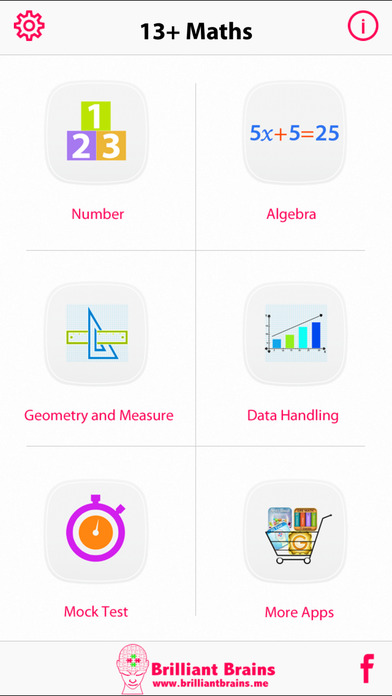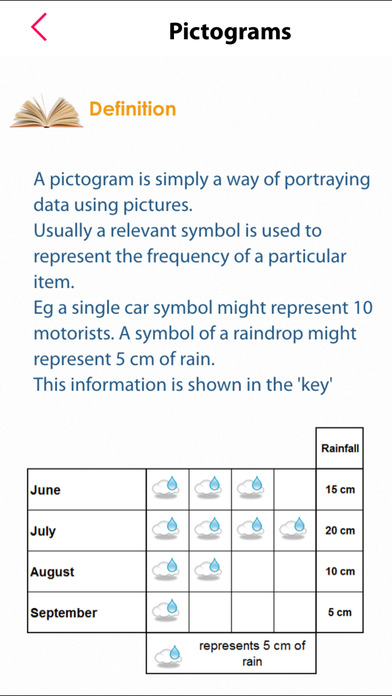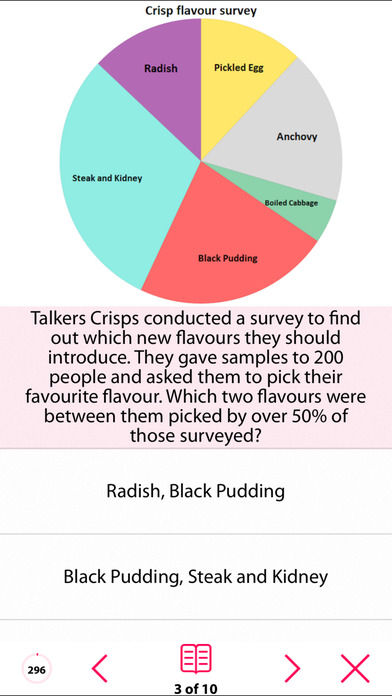# 13+ Maths Lite

## iOS UniversalEducation

Free

Over 140 revision notes and 1420 questions with explanations on Number, Algebra, Geometry & Measure and Data Handling.

DETAILED TEST RESULTS
On the completion of each test the user is given a summary of test along with the time taken on each question. Every question can be reviewed and all questions have an explanation of the answer.

PROGRESS METER
An interactive pie chart displays current progress for each topic so that you can track your weak areas and retry the questions you answered incorrectly.

MOCK TEST
Mock test simulates the real test format. Questions are presented randomly from all the topics.

REVISE BY SUBJECT

Number:

Approximation of calculations
Decimal places
Rounding
Significant figures
Addition & subtraction with negative numbers
Negative numbers
Number problems in words
Order of operations & BODMAS
Ordering numbers & place values
Factors
Highest common factor
Long division & multiplication
Lowest common multiple
Multiples
Multiplying & dividing by decimals & single-digit numbers
Multiplying & dividing with negative numbers
Prime factors
Prime numbers
Real-life problems using multiplication & division
Times tables
Adding & subtracting decimals & fractions
Calculating a percentage of a quantity
Converting decimals into fractions & vise-versa
Decimals - multiplication & division
Dividing fractions
Equivalent fractions
Equivalent percentages, fractions & decimals
Finding a fraction of a quantity
Fraction problems in words
Fractions of a shape
Improper fractions & mixed numbers
Multiplying fractions
One quantity as a fraction/percentage of another
Percentage change
Percentage problems in words
Calculated powers
Dividing & Multiplying powers
Dividing & Multiplying using powers of 10
Power of a power
Powers of 10
Square numbers
Square roots & cube roots
Standard form
Calculating with ratios
Density
Direct proportion
Dividing amounts in ratios
Ratio problems in words
Ratios & fractions
Speed

Algebra:

Equations with the variable on both sides
Expansion, simplification & factorisation
Formulae, expressions & equations
Inequalities on number lines
Inequalities
Multiplying expressions
Rearranging formulas
Setting up equations
Simplifying expressions
Simultaneous equations
Solving equations with brackets
Solving inequalities
Solving linear equations
Substitution
The language of algebra
Trial & improvement
Conversion graphs
Drawing graphs from tables
Negative co-ordinates
Finding the nth term from given patterns
Finding the nth term
Number sequences
Patterns in number
Special sequences
The nth term of a sequence

Geometry & Measure:

Area of a circle, rectangle & triangle
Area of compound shapes
Circumference of a circle
Constructions
Cuboids
Identifying 2D shapes
Parts of a circle
Perimeter
Polygons
Prisms
Pythagoras' theorem in real life
Pythagoras' theorem
Alternate angles
Angles & regular polygons
Angles around a point
Angles basics
Angles in a quadrilateral & triangle
Corresponding, Interior & Opposite angles
Converting measurements
Measuring time
Metric system
Nets
Plans & elevations
Units of length, area & volume
Congruent shapes
Reflections rotations & translations
Scaling & enlargements
Similarity
Symmetry

Data Handling:

Bar charts
Line graphs
Line of best fit
Pictograms
Pie charts
Scatter diagrams
Two way tables
Frequency tables
Mean, Median, Mode
Range
Addition rule for mutually exclusive outcomes
Calculating probabilities
Probability of not an event
The probability scale

FEATURE LIST

• Option to choose the number of questions to test.
• Option to choose if user wants instant feedback on answer selection.
• Progress meter helps to track progress, topic wise & mock test wise.
• Ability to re-attempt only the questions you get wrong or correct or never attempted
• Option to choose "ON" or "OFF" for sound effects.
• Option to choose reset the progress & start from scratch.

## What's New

Updated data and code to work with latest iOS version.

•••••